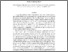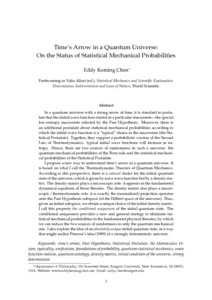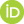# Time's Arrow in a Quantum Universe: On the Status of Statistical Mechanical Probabilities

Chen, Eddy Keming (2019) Time's Arrow in a Quantum Universe: On the Status of Statistical Mechanical Probabilities. [Preprint]Preview
Text
IPH-Prob 2.pdf

## Abstract

In a quantum universe with a strong arrow of time, it is standard to postulate that the initial wave function started in a particular macrostate---the special low-entropy macrostate selected by the Past Hypothesis. Moreover, there is an additional postulate about statistical mechanical probabilities according to which the initial wave function is a ''typical'' choice in the macrostate (the Statistical Postulate). Together, they support a probabilistic version of the Second Law of Thermodynamics: typical initial wave functions will increase in entropy. Hence, there are two sources of randomness in such a universe: the quantum-mechanical probabilities of the Born rule and the statistical mechanical probabilities of the Statistical Postulate.

I propose a new way to understand time's arrow in a quantum universe. It is based on what I call the Thermodynamic Theories of Quantum Mechanics. According to this perspective, there is a natural choice for the initial quantum state of the universe, which is given by not a wave function but by a density matrix. The density matrix plays a microscopic role: it appears in the fundamental dynamical equations of those theories. The density matrix also plays a macroscopic / thermodynamic role: it is exactly the (normalized) projection operator onto the Past Hypothesis subspace (of the Hilbert space of the universe). Thus, given an initial subspace, we obtain a unique choice of the initial density matrix. I call this property "the conditional uniqueness" of the initial quantum state. The conditional uniqueness provides a new and general strategy to eliminate statistical mechanical probabilities in the fundamental physical theories, by which we can reduce the two sources of randomness to only the quantum mechanical one. I also explore the idea of an "absolutely unique" initial quantum state, in a way that might realize Penrose's idea (1989) of a strongly deterministic universe.

 Export/Citation: EndNote | BibTeX | Dublin Core | ASCII/Text Citation (Chicago) | HTML Citation | OpenURL
 Social Networking:

Item Type: Preprint
Creators:
CreatorsEmailORCIDChen, Eddy Kemingeddyckm@gmail.com0000-0001-5144-0952
Additional Information: Forthcoming in Valia Allori (ed.), \emph{Statistical Mechanics and Scientific Explanation: Determinism, Indeterminism and Laws of Nature}, World Scientific
Keywords: time's arrow, Past Hypothesis, Statistical Postulate, the Mentaculus Vision, typicality, unification, foundations of probability, quantum statistical mechanics, wave function realism, quantum ontology, density matrix, initial condition of the universe, strong determinism
Subjects: General Issues > Determinism/Indeterminism
Specific Sciences > Probability/Statistics
Specific Sciences > Physics > Quantum Mechanics
Specific Sciences > Physics > Statistical Mechanics/Thermodynamics
Depositing User: Dr. Eddy Keming Chen
Date Deposited: 12 Feb 2019 15:22
Item ID: 15736
Subjects: General Issues > Determinism/Indeterminism
Specific Sciences > Probability/Statistics
Specific Sciences > Physics > Quantum Mechanics
Specific Sciences > Physics > Statistical Mechanics/Thermodynamics
Date: 11 February 2019
URI: http://philsci-archive.pitt.edu/id/eprint/15736

### Actions (login required)View Item